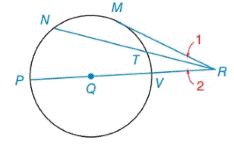Chapter 6.2, Problem 7EElementary Geometry For College St...

7th Edition
Alexander + 2 others
ISBN: 9781337614085

Solutions

Chapter
SectionElementary Geometry For College St...

7th Edition
Alexander + 2 others
ISBN: 9781337614085
Textbook Problem

Given: In ⊙ Q , P R ¯ contains Q, M R ¯ is a tangent, m M P ⌢ = 112 ° , m M N ⌢ = 60 ° and m M T ⌢ = 46 ° Find: a) m ∠ M R P b) m ∠ 1 c) m ∠ 2To determine

(a)

To find:

mMRP when Q, PR¯ contains Q, MR¯ is a tangent, mMP=112°, mMN=60° and mMT=46°.

Explanation

Theorem:

The measure of an angle formed by a tangent and a secant that intersect at a point outside the circle is one-half the difference of the measures of the two intercepted arc.

Calculation:

mMRP when Q, PR¯ contains Q, MR¯ is a tangent, mMP=112°, mMN=60° and mMT=46°

mMP+mMT+mTV=180°112°+46°+mTV=180°158°+mTV=180°m

To determine

(b)

To find:

m1 when Q, PR¯ contains Q, MR¯ is a tangent, mMP=112°, mMN=60° and mMT=46°.

To determine

(c)

To find:

m2 when Q, PR¯ contains Q, MR¯ is a tangent, mMP=112°, mMN=60° and mMT=46°.

Still sussing out bartleby?

Check out a sample textbook solution.

See a sample solution

The Solution to Your Study Problems

Bartleby provides explanations to thousands of textbook problems written by our experts, many with advanced degrees!

Get Started

What are the two requirements for a random sample?

Statistics for The Behavioral Sciences (MindTap Course List)

In Exercises 1728, use the logarithm identities to obtain the missing quantity.

Finite Mathematics and Applied Calculus (MindTap Course List)

If sin=47, find csc.

Trigonometry (MindTap Course List)

For f(x) = x2 and g(x) = 2x + 1, (f g)(x), = a) 2x2 + 1 b) (2x)2 + 1 c) (2x + 1)2 d) x2(2x + 1)

Study Guide for Stewart's Single Variable Calculus: Early Transcendentals, 8th

In Exercises 2532, find the inverse of the matrix if it exist. A=

Finite Mathematics for the Managerial, Life, and Social Sciences

Explain how a researcher using simple random sampling can still obtain a biased sample.

Research Methods for the Behavioral Sciences (MindTap Course List)